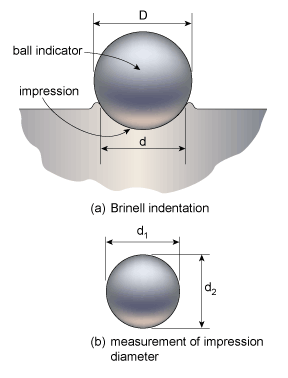# Brinell Hardness Principles

BRINELL HARDNESS PRINCIPLES

## Introduction to the Brinell Hardness

The Brinell hardness was studied and developed an Swedish engineer Johan August Brinell, and proposed it in 1900. They carry out the test by pressing a ball indenter against the surface of the specimen with a standard force. A certain ratio of diameter/force will create a certain indentation.

### Brinell Hardness Principles

Steel ball diameter can be 10mm or 5mm or 2.5mm or 1mm, the load can be from 6.25kgf to 3000kgf. The load P relative to the diameter D is equal to the ratio P/D2 (like 30:1; 15:1; 5:1; 2:1; 1.25:1; 1:1), which is normalized for different materials for accurate and stable test results. For steel material, the ratio is 30:1, for example, 10 mm ball indenter can use 3000 kgf force, or 1 mm ball indenter can use 30 kgf force. For example aluminum alloy, this ratio is 5:1. They keep the fixed acceleration for a period of time, usually 30 seconds. When the probe lifts up, they measure the diameters d1 and d2 of the indentation by using a microscope with a calibrated internal grid.#### They calculate the Brinell hardness (BHN) as the force divided by the surface area of the indent:#### Where:

• P = applied load in kilogram-force (kgf)
• D = diameter of indenter (mm)
• d = diameter of indentation (mm)

Sometimes, they quote Brinell hardness in megapascals. They multiplied the Brinell hardness number by the acceleration due to gravity, 9.80665 m/s2, to convert it to megapascals.

They can convert the BHN into the Ultimate Tensile Strength (UTS), although the relationship is dependent on the material, and therefore determined empirically. The relationship is based on Meyer’s index (n) from Meyer’s law. If Meyer’s index is less than 2.2 then the ratio of UTS to BHN is 0.36. If Meyer’s index is greater than 2.2, then the ratio increases.

To measure BHN, the people designate the most commonly used test standards (ASTM E10-14 and ISO 6506–1:2005) as HBW (H from hardness, B from Brinell, and W from the material of the indenter, tungsten (wolfram) carbide). In former standards, the people measured the HB or HBS with steel indenters.

They calculate HBW in both standards using the SI units as:• F = applied load (newtons).
• D = diameter of indenter (mm).
• d = diameter of indentation (mm).

Normally special tables are used in practice. These tables give numerical values of Brinell hardness for different indentation diameters and obtained under different loads when using different diameters of the balls.

To measure the diameter of the indent, the people use a microscope with magnification X20 or X40.

#### Notes on the Brinell scale

Please note that to obtain good results in the determination of Brinell hardness, the following rules should be observed:

• Load should be applied in a direction strictly perpendicular to the plane of the test specimen;
• The indentation center should be located at a distance of not less than two diameters of the ball from the end of the specimen;
• The indentation diameter should be measured in two mutually perpendicular directions;
• The diameter of the indentations resulting from the tests should be within a range of 0.2D to 0.6D.

Normally, this method is not employed for testing materials with a hardness of more than 450, as in this case the test results are characterized by a large scatter. The Rockwell diamond cone or Vickers test methods are usually used for these purposes.

## Hardness testing machine

### Brinell hardness tester

#### You may also like...

error: Content is protected !!